## Monday, October 23, 2006

### A Generating Function for the Bernoulli Numbers

In my previous blog, I defined the Bernoulli numbers using a recurrence relation:

In today's blog, I will show how the Bernoulli numbers can be used with a generating function. A generating function is a power series, that is, a compact expression that defines an infinite sum. For background on generating functions, I recommend the wikipedia article (see reference) or Graham et al's Concrete Mathematics (see reference).

The content in today's blog is taken from Concrete Mathematics. The term "binomial convolution" is taken from this work. A "convolution" is the multiplication of two generating functions. This lemma is called a "binomial convolution" by Graham et al because the result involves binomial coefficients (see binomial theorem, here, for more details).

Lemma 1: Binomial Convolution

Let: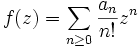h(z) = f(z)*g(z)

Then there exists dn such that:with: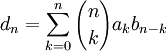Proof:

(1) Multiplying f(z) with g(z) gives us: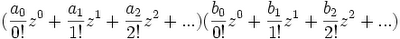(2) Grouping the result by zi gives us: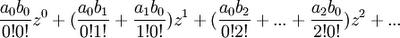(3) If we set ci to each coefficient. For example, if we set c0 to (a0b0)/(0!0!), then we have the following formula:(4) Now, let's define a value di such that:(5) This then gives us that:
di/(i!) = ci

(6) Combining step #5 with step #2 and step #3 gives us:QED

Thereom: Generating Function for the Bernoulli Numbers

z/(ez - 1) is the generating function for the Bernoulli numbers

Proof:

(1) Let G(z) = ∑ (n ≥ 0) Bnzn/n! where Bi is the Bernoulli number for i.

(2) We know from a previous result (see Lemma 2, here) that:

ez = ∑ (n ≥ 0) zn/n!

(3) So we can use Lemma 1 above to show that: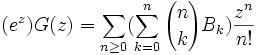(4) Now, the definition for Bernoulli numbers (see Definition 1, here) is: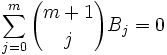for all cases except for B0 where B0 = 1.

(5) If we let n=m+1 and add Bn to both sides, then we have: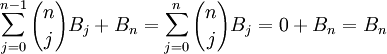or Bn + 1 in the case where n=m+1=1.

(6) This enables us to simplify the result in step #3 to get: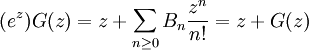Note: the z at the bottom comes from the fact that at n=1, our result is (B1+1)z1/1! = B1z1/1! + z.

(7) If we subtract G(z) from both sides, we get:

(ez)G(z) - G(z) = z =

G(z)(ez - 1) = z

(8) Dividing both sides by ez - 1 gives us:

G(z) = z/(ez - 1)

QED

Corollary: B2i+1=0 if i ≥ 1.

Proof:

(1) z/(ez - 1) = ∑ (n ≥ 0) Bnzn/n! [This follows from the theorem above]

(2) Now, B1 = -(1/2) [See Definition 1, here]

(3) So,

z/(ez - 1) + (z/2) = ∑ (n ≥ 0 and n ≠ 1) Bnzn/n!

We subtract (-z/2) instead of -1/2 because we are removing this item from a generating function where each value in the sum z/(ez-1) pairs up with a unique term of the sum.

In this case, B1 matches up with the term B1(z1)/1! which equals -z/2.

(4) z/(ez - 1) + (z/2) = (2z + z[ez - 1])/(2[ez-1]) = (2z -z + z[ez])/(2[ez-1]) =

= (z/2)(e
z+1)/(ez - 1)

(5) (z/2)(ez + 1)/(ez-1) = [e-(z/2)/e-(z/2)]* (z/2)(ez + 1)/(ez-1) =

= (z/2)[(ez/2 + e-z/2)/(ez/2 - e-z/2)]

(6) So, we see in step #5, that if we replace z with (-z), the identity remains unchanged.

(-z/2)[(e-z/2 + e-(-z/2)]/(e-z/2 - e-(-z)/2) =

= -z/2[e-z/2 + ez/2]/(e-z/2 - ez/2) =

= z/2[ez/2 + e-z/2]/(ez/2 - e-z/2)

In the language of mathematics, a function is called even if f(-x)=f(x) and we call a function odd if f(-x) = -f(x). [See here for more details]

Example: cos(x) is an even function while sin(x) is an odd function. [See here for review of sin and cos]

(7) But this means that the same must hold true for ∑ (n ≥ 0 and n ≠ 1) Bnzn/n!

Since by Step #3, we showed that they are equal functions.

So that we have:

∑ (n ≥ 0 and n ≠ 1) Bnzn/n! = ∑ (n ≥ 0 and n ≠ 1) Bn(-z)n/n! = ∑ (n ≥ 0 and n ≠ 1) (-1)n(Bnzn/n!)

(8) Matching up each of the terms according to powers of zn, this gives us that:

Bn = (-1)nBn

(9) But this can only be true if Bn = 0 when n is odd.

QED

References

#### 4 comments:

astifter said...

Hi,

I really don't get the equation 4 in the proof of Lemma 1. Can anyone please explain? (Or point me to an explanation?)

Thanks, astifter

Larry Freeman said...

Hi Astifter,

Thanks very much for your question. I've rewritten the first lemma in order to make it clearer.

Please let me know if you still have questions.

Cheers,

-Larry

st said...

I do not get the note below step 6. (Note: the z at the bottom comes from the fact that at n=1, our result is (B1+1)z1/1! = B1z1/1! + z.)

Kazbich-the-bandit said...

st, there is a simple explanation and it has to do with the fact that B0 = 1. I dont have the right mathematical symbols so bear with me - S means sum and bi () is for the binomial coefficient:
S(k=0,n) bi(n+1 k) Bk = 0 is valid for all n except for n=0 where it is equal to 1. Now consider
S (n=0, infinity) [S (k=0, n) bi(n k) Bk)] z^n/n! and expand it up to n=2:
So for n = 0, we have the first term = 1
for n = 1, we have 2nd term :(B0 + B1)z
for n = 2, we have 3rd term (B0 + 2B1 + B2)(z^2/2!)
But B0 + 2B1 = 0 since this is equal to S (k=0, 1) bi(2 k)Bk
Therefore if we add all terms up to
n= 2, remembering that B0 = 1 we get
1 + z + zB1 + z^2/2!B2
Now do the same with the sum in the result in step 6:
S (n=0, infinity) Bnz^n/n! up to n=2: you should get
1 + B1z + B2z^2/2!
with a missing z because Bo is not equal to zero.
I hope I have managed to shed some light:)
Thanks for the page, Larry, I've been looking for something like this for ages.